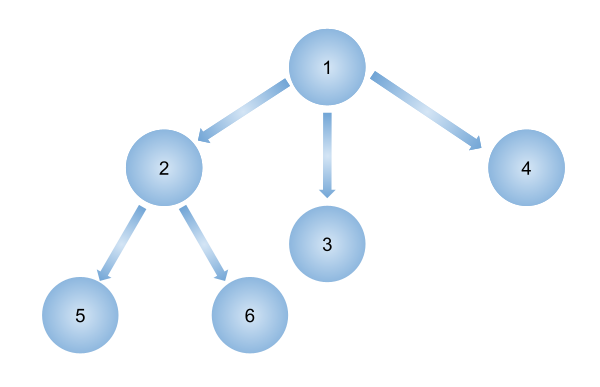# Serialize and Deserialize an N-ary tree

Posted: 27 Mar, 2021
Difficulty: Hard

## PROBLEM STATEMENT

#### In simple words, serialization is a process to store a tree in a file so that it can be later restored to its original form. Deserialization is reading the tree back from the file.

##### Note:
``````Note that the structure of the tree must be maintained while serialization and deserialization. Also, you can use any method to serialize or deserialize the tree. You just need to see that the tree can be serialized into a string and further deserialized into the original tree.
``````
##### Input Format:
``````The first line contains an integer 'T' which denotes the number of test cases or queries to be run. Then the test cases are as follows.

The first line of each test case contains elements of the N-ary tree in the level order form. The line consists of values of nodes separated by a single space. In case a node is changed, we take -1. To get the next node

The first not-null node(of the previous level) is treated as the parent of the first nodes of the current level. The second not-null node (of the previous level) is treated as the parent node for the next nodes of the current level and so on.

The input ends when all nodes at the last level are null(-1).
``````
##### Note :
``````The above format was just to provide clarity on how the input is formed for a given tree.
The sequence will be put together in a single line separated by a single space. Hence, for the below-depicted tree, the input will be given as:
1 -1 2 3 4 -1 5 6 -1 -1 -1 -1 -1
``````##### Output Format:
``````For each test case, you need to print the deserialized tree after its serialization.

Print the output of each test case in a separate line.
``````
##### Note:
``````You don’t need to print anything; It has already been taken care of. Just implement the given function.
``````
##### Constraints
``````1 <= T <= 10
1 <=  N <= 10^4

Time limit: 1 sec
``````Approach 1

Serialization - In serialization, we have to convert the tree into serial order. So we will put the tree node’s value in a vector in such a way that after every node’s child values we will append a ‘-1’.

We will maintain a queue that will store tree nodes. For every node, we will push its child node’s value in the vector and push its child nodes in the queue so that they can be explored further. After pushing all the child node’s value, we will push ‘-1’ to the vector. We will continuously explore all the nodes until the queue is not empty.

Algorithm

1. Serialization

• Let ‘FILE’ be the vector/list that will store the serial order of the tree.
• Create a queue ‘Q’ that will store nodes of the tree.
• If the ROOT is NULL, return.
• Push DATA of the ROOT in ‘FILE’ and ‘ROOT’ to queue.
• Start traversing until the queue is not empty.
• Store the front node of the queue in “CURRENT_NODE” and pop out this front node.
• Push all the child node’s value of “CURRENT_NODE” in 'FILE'
• Push all the child nodes of “CURRENT_NODE” to ‘Q’
• After pushing all the child values, push ‘-1’ to “FILE”.

2. Deserialization

For deserialization, we have to convert the values in the vector ‘FILE’ to an N-ary tree. We will take a queue as we have maintained in serialization and initially push a node with data equal to FILE[ 0 ]. As we have appended ‘-1’ after child nodes of every node. So all the nodes before ‘-1’ will become child nodes of the node at the front of the queue.

So we will create nodes until ‘-1’ is encountered. All these nodes will be the child of the node at front of the queue and we will push all these nodes to ‘Q’ so that we can get the child nodes of these nodes also.

Algorithm

• Create a queue ‘Q’ that will store nodes of the tree.
• If FILE[ 0 ] = -1, i.e there is no root node, return NULL.
• Create a node ‘ROOT’ with data value = FILE[ 0 ].
• Push this ‘ROOT’ node to the queue.
• Take a variable ‘INDEX’ that will store the index of ‘FILE’ that we have to deserialize. (initialized to 1)
• Traverse through ‘Q’ until ‘Q’ is not empty.
• Store the front node of the queue in “CURRENT_NODE” and pop out this front node.
• Take a vector “CHILDREN” that will store all the child nodes of the ‘CURRENT_NODE’.
• Now traverse through ‘FILE’ from ‘INDEX’ until ‘-1’ is not encountered.
• Create nodes with values FILE[ INDEX ] and push it to ‘CHILDREN’ and ‘Q’.
• Store this ‘CHILDREN’ vector to the child of “CURRENT_NODE”